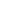top
Stepper Motor System• Easy Control with Pulse Signals

A system configuration for high accuracy positioning is shown below. The rotation angle and speed of the stepping motor can be controlled accurately using pulse signals from the controller.• What is a Pulse Signal?

A pulse signal is an electrical signal whose voltage level changes repeatedly between ON and OFF.
Each ON/OFF cycle is counted as one pulse. A command with one pulse causes the motor output shaft to turn by one step. The signal levels corresponding to voltage ON and OFF conditions are referred to as "H" and "L," respectively.• The length of Rotation is Proportional to the Number of Pulses

The length of rotation of the stepping motor is proportional to the number of pulse signals (pulse number) given to the driver. The relationship of the stepper motor's rotation (rotation angle of the motor output shaft) and pulse number is expressed as follows:• The Speed is Proportional to the Pulse Frequency

The speed of the stepper motor is proportional to the frequency of pulse signals given to the driver.
The relationship of the pulse frequency [Hz] and motor speed [r/min] is expressed as follows:wechat QR codeChat with robot Hermione